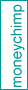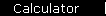### Fibonacci Numbers in Finance

Fibonacci numbers are a sequence where each term equals the sum of the previous two:

1,  1,  2,  3,  5,  8,  13,  21,  34,  55, . . .

The "34", for example, belongs in the sequence because it equals 13  +  21.

A second property is that as you go out in the sequence, the ratios of neighboring terms approach a fixed ratio of about 1.618:

 Terms:

Fibonacci numbers seem to occur in nature, and it has even been suggested that our brains are hardwired to find Fibonacci progressions naturally pleasing. Where they show up in investing is in technical analysis, where "the fibs" are supposed to predict the behavior of the stock market. The theory is that the collective "investor mind" must also be hardwired so that interesting things happen at Fibonacci-sized intervals.

Believe it or not, there really is a connection between Fibonacci numbers and finance; but once you see what it is, it tends to make the whole thing seem sort of banal. That second property, that the sequence grows at a fixed ratio, also means that it grows almost exactly like compound interest:

 The Fibs   Compound Interest Terms:Here the "return rate" between the bars is 61.8%; but throw in a scaling factor you can turn it into any compound interest curve you want. What this means is that you can take something obvious, like investments growing like compound interest over the long run, and then make it seem mysterious and intriguing by trying to explain it in terms of Fibonacci numbers... ...89,  144,  233... ...Finally, the thing at the left is a "Fibonacci tiling"; the squares spiral out counter-clockwise from the red one in the center, and have sides of length 1, 1, 2, 3, 5, 8... Some people say that if you stare at this figure long enough your brain will jiggle.

 home  |  features  |  glossary  |  calculator  |  about us  |  books